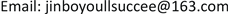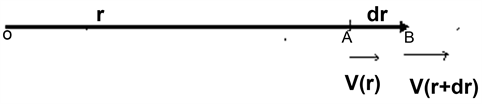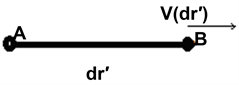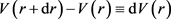﻿ 星体的速度与距离之关系 The Relationship of Velocity and Distance of Stars

Modern Physics
Vol. 09  No. 03 ( 2019 ), Article ID: 30065 , 4 pages
10.12677/MP.2019.93015

The Relationship of Velocity and Distance of Stars

Kaige Jin*

Xuzhou JiangsuReceived: Apr. 9th, 2019; accepted: Apr. 28th, 2019; published: May 5th, 2019ABSTRACT

This paper which proceeds from the principle of superposition of velocity in Special Relativity has made a relationship between Stellar Velocity and its distance.

Keywords:Hubble’s Law, Stellar Velocity, Special Relativity1. 哈勃定律的局限

$z=\frac{H}{C}r$ (1.1)

$\frac{\omega }{{\omega }_{0}}=\sqrt{\frac{1+V/c}{1-V/c}}$ (1.2)

$z=\frac{\omega -{\omega }_{0}}{\omega }\approx \frac{V}{C}$ (1.3)

$V=Hr$ (1.4)

H称作哈勃常数，其倒数具有时间的量纲，常常认为是宇宙的年龄，约为140亿年左右。

2. 星体速度与距离的关系

$V=V\left(r\right)$ (2.1)Figure 1. Distance A is R and distance B is r + dr with o as observation pointFigure 2. A as the observation point

$V\left(\text{d}{r}^{\prime }\right)=H\text{d}{r}^{\prime }$ (2.2)

$V\left(r+\text{d}r\right)=\frac{V\left(r\right)+V\left(\text{d}{r}^{\prime }\right)}{1+\frac{V\left(r\right)V\left(\text{d}{r}^{\prime }\right)}{{C}^{2}}}=\frac{V\left(r\right)+H\left(\text{d}{r}^{\prime }\right)}{1+\frac{V\left(r\right)H\left(\text{d}{r}^{\prime }\right)}{{C}^{2}}}$

$\begin{array}{l}V\left(r+\text{d}r\right)=\frac{V\left(r\right)+H\text{d}{r}^{\prime }}{1+\frac{V\left(r\right)H\text{d}{r}^{\prime }}{{C}^{2}}}\approx \left(V\left(r\right)+H\text{d}{r}^{\prime }\right)\left(1-\frac{V\left(r\right)H\text{d}{r}^{\prime }}{{C}^{2}}\right)\\ \text{\hspace{0.17em}}\text{\hspace{0.17em}}\text{\hspace{0.17em}}\text{\hspace{0.17em}}\text{\hspace{0.17em}}\text{\hspace{0.17em}}\text{\hspace{0.17em}}\text{\hspace{0.17em}}\text{\hspace{0.17em}}\text{\hspace{0.17em}}\text{\hspace{0.17em}}\text{\hspace{0.17em}}\text{\hspace{0.17em}}\text{\hspace{0.17em}}=V\left(r\right)+H\text{d}{r}^{\prime }-\frac{V{\left(r\right)}^{2}H\text{d}{r}^{\prime }}{{C}^{2}}-\frac{V\left(r\right){H}^{2}{\left(\text{d}{r}^{\prime }\right)}^{2}}{{C}^{2}}\end{array}$

$V\left(r+\text{d}r\right)-V\left(r\right)=H\text{d}{r}^{\prime }-\frac{V{\left(r\right)}^{2}H\text{d}{r}^{\prime }}{{C}^{2}}=\left(1-\frac{V{\left(r\right)}^{2}}{{C}^{2}}\right){H}_{0}\text{d}{r}^{\prime }$ (2.3)

dr′与dr的关系满足

$\text{d}{r}^{\prime }=\gamma \text{d}r$ (2.4)

$\gamma =\frac{1}{\sqrt{1-\frac{{V}^{2}}{{C}^{2}}}}$$\frac{\text{d}V}{\sqrt{1-\frac{{V}^{2}}{{C}^{2}}}}=H\text{d}r$ (2.5)

$V=C\mathrm{sin}\left(\frac{Hr}{C}\right)$ (2.6)

3. 结论与问题

3.1. 条件限制

1) 公式(2.6)的导出，隐含了宇宙是均匀的各向同性的宇宙学原理。在没有考虑宇宙在大尺度上的各向异性。

2) 假定了宇宙的近似平坦，这个假定与宇宙膨胀似乎不符，近似程度较难确定。

3.2. 公式的普适性

1) 近距离时，(2.6)可以回到哈勃定律。但近距离星体的本动有可能是决定性的，导致星体退行速度的测量值与公式可能有更大的偏差，不过，考虑到如果把哈勃定律适用的尺度作为距离的微元，有其合理的因素。

2) 当观察距离

$r1=\frac{\pi C}{2{H}_{0}}$ 时，

3) 当

$r2=\frac{\pi C}{{H}_{0}}$

The Relationship of Velocity and Distance of Stars[J]. 现代物理, 2019, 09(03): 126-129. https://doi.org/10.12677/MP.2019.93015

1. 1. 俞允强. 物理宇宙学讲义[M]. 北京: 北京大学出版社, 2002: 57, 72, 121.

2. 2. Kazantzidis, L., Perivolaropoulos, L. and Skara, F. (2019) Constraining Power of Cosmological Observables: Blind Redshift Spots and Optimal Ranges. Physical Review D, 99, Article ID: 063537.
https://doi.org/10.1103/PhysRevD.99.063537

3. NOTES

*通讯地址：徐州西苑民安园36-1-104。